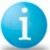# Confusion in Yield, DPMO, DPU

Six Sigma – iSixSigma Forums Old Forums General Confusion in Yield, DPMO, DPU

Viewing 6 posts - 1 through 6 (of 6 total)
• Author
Posts
• #28418

Hi,
I am having a lot of confusion in the following six sigma metrcis.
First Pass Yield, Rolled throughput Yield, DPU, DPMO.  How to calculated these metrics, and how to convert them into sigma level. Please put some light on these metrics.
thanks
sridhar

0
#70759Jim Johnson
Participant

I am going to answer your question in individual parts.First Time Yield (FTT) is simply the number of good units produced divided by the number of total units going into the process. For example:You have a process of that is divided into four sub-processes – A, B, C and D. Assume that you have 100 units entering process A. To calculate FTT you would:1. Calculate the yield (number out of step/number into step) of each step.
2. Multiply these together.For Example:100 units enter A and 90 leave. The FTT for process A is 90/100 = .990 units go into B and 80 units leave. The FTT for process B is 80/90 = .8980 units go into C and 75 leave. The FTT for C is 75/80 = .9475 units got into D and 70 leave. The FTT for D is 70/75 = .93The total process yield is equal to FTTofA * FTTofB * FTTofC * FTTofD or .9*.89*.94*.93 = .70.You can also get the total process yield for the entire process by simply dividing the number of good units produced by the number going in to the start of the process. In this case, 70/100 = .70 or 70 percent yield.I hope that this helps. Jim Johnson

0
#70760Jim Johnson
Participant

Next we will turn our attention to Rolled Throughput yield. If you will remember, the First Time Yield calculation we did (FTT) considered only what went into a process step and what went out. Rolled Throughput Yield adds the consideration of rework. Using the previous example:Process A = 100 units in and 90 out
Process B = 90 in and 80 out
Process C = 80 in and 75 out
Process D = 75 in and 70 out.If in order to get the yield out of each step we had to do some rework (which we probably did) then it really looks more like this:Process A = 100 units, 10 scrapped and 5 reworked to get the 90. The calculation becomes 100-(10+5)/100 = 85/100 = .85 This is the true yield when you consider rework and scrap.Process B = 90 units in, 10 scrapped and 7 reworked to get the 80. 90-(10+7)/90 = .81Process C = 80 units in, 5 scrapped and 3 reworked to get the 75. 80-(5+3)/80 = .9Process D = 75 units in, 5 scrapped and 10 reworked to get the 70. 75-(5+10)/75 = .8Now to get the true Rolled Throughput Yield (Considering BOTH scrap and the rework necessary to attain what we thought was first time througput yield) we find that the true yield has gone down significantly:.85*.81*.9*.8 = .50 or 50% yield. A substantially worse and substantially truer measurement of the process capability.

0
#70761Jim Johnson
Participant

DPU or Defects Per Unit is simply as the nomenclature would suggest. How many defects can we expect to find in a single unit based on our process capability? Consider 100 loan applications. If out of the 100 loans applications there are 30 defects, the FTT yield is .70 or 70 percent. Further investigation finds that 10 of the 70 had to be reworked to achieve that yield so our Rolled Throughput Yield is 100-(30+10)/100 = .6 or 60 percent yield.To consider the defects per unit in this process we divide the number of defects by the result of multiplying the sample by the number of opportunities in each item.30/100*3 = 30/300 = .1 or we would say that there is a 10 percent chance for a defect to occur in this process.Now to convert the DPU to DPMO we simply multiply the DPU by 1,000,000. This would tell us that out of every 1,000,000 loan applications that we processed we would expect to see 100,000 defects. So what is the difference? In calculating FTT and RTTY we are counting the number of defectives (including the defectives that were repaired in the process). In calculating DPU and DPMO we are focusing on the number of defects. Remember that a loan application has three opportunities to be defective. A given defective application could have one, two or three defects to qualify. DPU and DPMO consider this, while yield calculations do not.I hope that this helps.Jim Johnson

0
#70795Ravi Khare
Participant

Thanks Jim,
The simply worded explaination certainly helps. I too had some confusion in my mind.
Ravi Khare

0
#70801Member

Dear Jim,